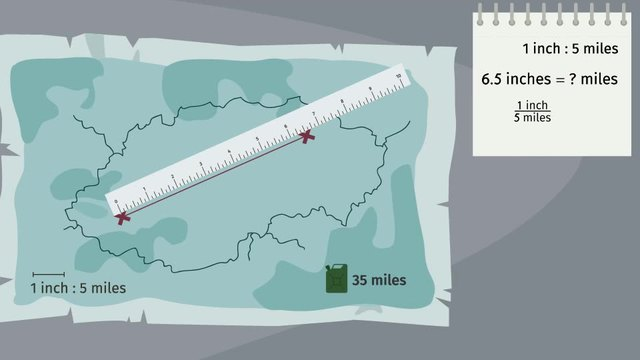# Computing Actual Lengths from a Scale DrawingRating

Ø 5.0 / 1 ratings

The authorsTeam Digital

## Basics on the topicComputing Actual Lengths from a Scale Drawing

After this lesson, you will be able to compute actual lengths represented in a scale drawing by using the scale ratio rather than the scale factor.

The lesson begins by teaching you the difference between a scale and scale factor. It leads you to setting up a proportion using the scale ratio as a fraction. It concludes with solving proportions for an unknown number using cross multiplication.

Learn about computing lengths using scale ratios by helping Frank find Adventure Mike in Siberia!

This video includes key concepts, notation, and vocabulary such as the term scale or scale ratio (a type of ratio in which the units are mixed, ie. Inches to miles, cm to km); how to read scale ratio notation (1 cm represents 20 m or 1:20 which is read 1 to 20); and the term scale factor (the constant of proportionality k in the equation y=kx where x is a length in the original image and y is the length in the scaled image).

Before watching this video, you should already be familiar with scale factors, multiple units of measurement (metric and imperial units), and cross-multiplication.

After watching this video, you will be prepared to learn to compute the actual areas represented by scale drawings.

Common Core Standard(s) in focus: 7.G.A.1 A video intended for math students in the 7th grade Recommended for students who are 12 - 13 years old